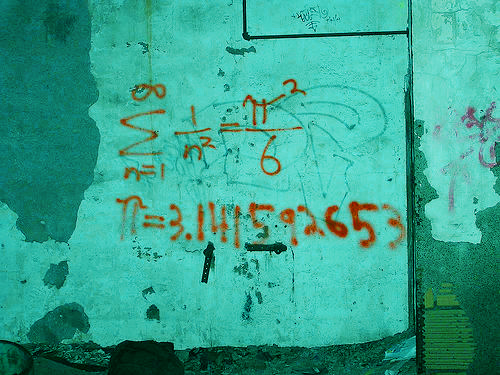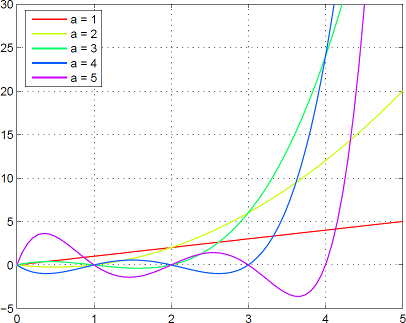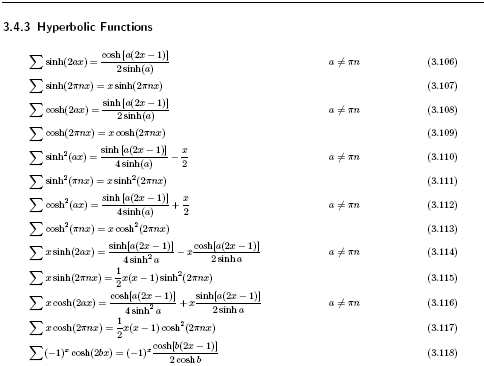# Difference and Functional Equations ReferenceOriginal image by openDemocracy.

The document below contains tables and formulas useful for working with functional equations, especially difference equations, and to a lesser extent, quotient equations (where differences are replaced by quotients).

The reference contains tables for forward differences, (indefinite) sums, quotients, and products. There is also a table of z-transforms, binomial transfroms, formulas for converting certain kinds of functional equations to difference equations and some discrete Taylor series. There are more than 500 formulas in its 65 pages.

This is a work in progress, so be sure to read the preface (which highlights some of the issues with this document). If you find any errors, please comment below.## Changes

### Version 1.1

• Added Exponential Sums to differences and sums.
• Additions to the z-transform table.

### Version 1.2

• Expanded the section on the discrete power functions.
• Expanded the section that explain the sue of constants in the table.
• Added forms involving the following expressions to the sum (x + h) tables:
• $ax + b$
• $x^2 + a^2$
• Updated all the graphs, and added some new ones.
• Reorganized slightly, and fixed some typos.
• Added a few examples, explanations, and additional notations in the sum ($x + h$) tables.

### Version 1.3

• Added the chain and substitution rules for arithmetic differences.
• Added table of functions for reference.
• Expanded the introduction somewhat.
• Added definition for arithmetic difference analogs.
• Added rules for manipulating arithmetic difference analogs.
• Added several new entries, including several functions whose sums can be expressed as the sum of $E(x) = 1/(eix + 1)$.
• Expressed the G-function (sum of the Gamma function) as a product of known functions, and replaced its notation. The notation G(x) is now used for the Barnes G-function.

### Version 1.4

• Made, as always, a few corrections.
• Added the tangent sum function. There are still many details to sort out for this and related functions (cot, sec, csc, their hyperbolic counterparts, $1/(ex+1)$, and so on), and hence these sections are still messy. These will be cleaned up as the details become clear.
• Replaced some of the statements on periodic, odd, and even functions with precise versions. The previous ones were only correct up to a periodic function.
• Added the derangement function (expressed in terms of the incomplete gamma function), as well as some related Taylor series.
• Since I included the definitions of analog functions, I discovered that the intuitive notion of analogs did not correspond to the definition. Thus, the analogs of ln x and atan x have been removed / replaced. These might re-appear if the definition of analog functions is suitably adjusted.
• Made many statements on the z-transform more precise.
• Made some notations more consistent with standard notation.

### Version 1.5

• Made a small correction for the binomial law for discrete powers.

DiscreteCalculusTables_1_5 (PDF 4.6 MB).

1.Caves says:
2.herman.tulleken says: